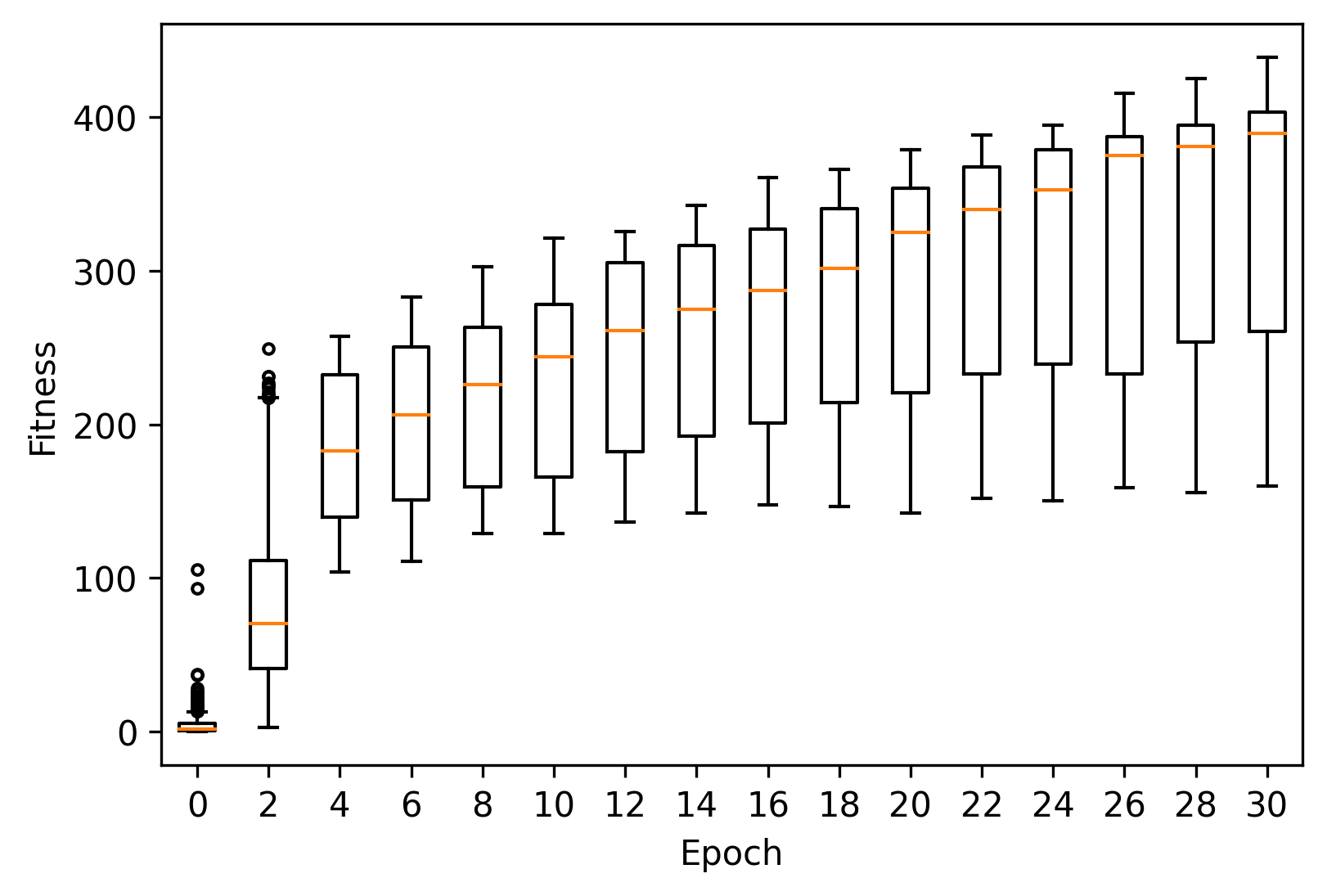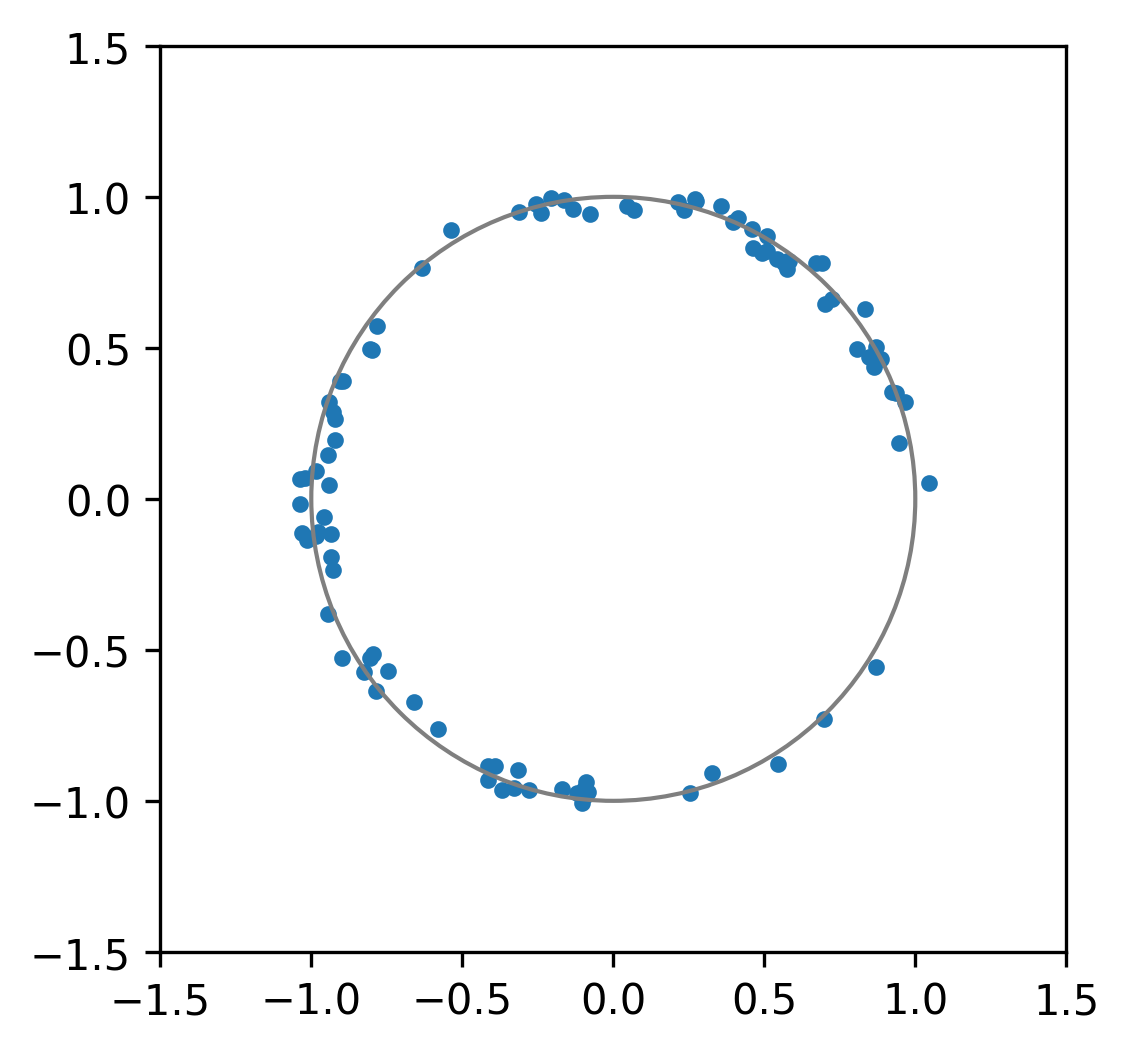# Simulating the unit circle¶

Consider the following scenario. Let $$X$$ be a dataset with two columns denoted by $$X_r$$ and $$X_a$$ respectively, each containing $$n \in [50, 100]$$ values. The values of $$X_a$$ are drawn from the interval $$[-2\pi, 2\pi]$$ while those in $$X_r$$ may take values from $$[0, 2]$$.

Our aim is to find a dataset $$X$$ that maximises the following function:

$f(X) = \frac{\text{Var}\left(X_{a}\right)}{\max_{x \in X_r} \left|x - 1\right|}$

That is, a dataset which maximises the variance of one column and minimises the maximal distance from one of the other.

Such a dataset would describe the polar coordinates of some set of points along the unit circle where the points in $$X_r$$ corresponds to the radii and those in $$X_a$$ correspond to the angle from the origin in radians.

## Formulation¶

For the sake of ease, we will assume that each column of $$X$$ can be uniformly distributed between the prescribed bounds.

Then, to formulate this scenario in edo, we will have to create some copies of the Uniform class.

:

import edo
import numpy as np
import pandas as pd
from edo.distributions import Uniform

:

class RadiusUniform(Uniform):

param_limits = {"bounds": [0, 2]}

class AngleUniform(Uniform):

name = "AngleUniform"
param_limits = {"bounds": [-2 * np.pi, 2 * np.pi]}


To keep track of which column is which when calculating the fitness of an individual, we will use a function to extract that information from the metadata.

:

def split_individual(individual):
""" Separate the columns of an individual's dataframe. """

names = [m.name for m in metadata]
angles = df[names.index("AngleUniform")]

def fitness(individual):
""" Determine the similarity of the dataset to the unit circle. """

return angles.var() / (radii - 1).abs().max()


Given that this is a somewhat more complicated task than the previous tutorial, we will employ the following measures:

• A smaller proportion of the best individuals in a population will be used to create parents
• The algorithm will be run using several seeds to explore more of the search space and to have a higher degree of confidence in the output of edo
:

pop_histories, fit_histories = [], []
for seed in range(5):

opt = edo.DataOptimiser(
fitness,
size=100,
row_limits=[50, 100],
col_limits=[(1, 1), (1, 1)],
families=families,
max_iter=30,
best_prop=0.1,
maximise=True,
)

pops, fits = opt.run(random_state=seed)

fits["seed"] = seed
pop_histories.append(pops)
fit_histories.append(fits)

fit_history = pd.concat(fit_histories)


## Visualising the results¶

As before, we can plot the fitness progression across these runs using matplotlib.

:

import matplotlib.pyplot as plt

:

_, ax = plt.subplots(dpi=300)

epochs = range(0, 31, 2)
fit = fit_history[fit_history["generation"].isin(epochs)]

xticklabels = []
for pos, (gen, data) in enumerate(fit.groupby("generation")):
ax.boxplot(data["fitness"], positions=[pos], widths=0.5, sym=".")
xticklabels.append(gen)

ax.set_xticklabels(xticklabels)
ax.set_xlabel("Epoch")
ax.set_ylabel("Fitness")

:

Text(0, 0.5, 'Fitness')We can also take a look at the best individual across all runs.

:

idx = fit_history["fitness"].idxmax()
seed, gen, ind = fit_history[["seed", "generation", "individual"]].iloc[idx]

best = pop_histories[seed][gen][ind]
seed, gen, ind, best

:

(0,
30,
49,
Individual(dataframe=           0         1
0   1.005690 -4.928099
1   0.971288  1.524298
2   1.000057 -5.924903
3   0.941059  2.931472
4   0.984278  3.252393
..       ...       ...
89  0.989021  4.615850
90  1.022580  3.276894
91  0.992919  3.266881
92  1.027503 -4.978339
93  1.004182  4.431548

[94 rows x 2 columns], metadata=[Uniform(bounds=[0.93, 1.05]), Uniform(bounds=[-6.0, 4.74])]))

:

_, ax = plt.subplots(dpi=300)

circle = plt.Circle((0, 0), 1, fill=False, linestyle="-", color="tab:gray")

scatter = ax.scatter(xs, ys, marker=".")

ax.set(xlim=(-1.5, 1.5), ylim=(-1.5, 1.5), aspect="equal")

:

[(-1.5, 1.5), (-1.5, 1.5), None]That’s a pretty good approximation to a circle. Nice.

Generated by nbsphinx from a Jupyter notebook. Formatted using Blackbook.